# VLOOKUP NamesArticle byJeevan A Y## What Is VLOOKUP Using Names?

VLOOKUP Names helps us lookup for a value from a particular named range. If you are familiar with the VLOOKUP function in Excel, you must know that we must select the table array to specify the VLOOKUP function to look for the desired value in that table range.

Sometimes when we work with large amounts of data, It gets frustrating to select a large data range. If the data range is in different worksheets, then switching between the worksheets to select the data range is time-consuming. This is where the concept of ‘VLOOKUP Names’ or ‘Named Ranges’ comes in handy.

Irrespective of which worksheet we are in, if the data range is a named range, then we can use that name to refer to that data table with ease. So, users don’t have to toggle between worksheets or scroll through a large data range to choose the table array.

###### Key Takeaways
• VLOOKUP Names helps us lookup for a value in a named range.
• By using a named range, we can eliminate the risk of choosing the wrong data range or eliminate the manual process of scrolling through ranges or worksheets.
• The dynamic named ranges will take new additional rows and columns into consideration automatically, and VLOOKUP will retrieve the values for the newly added lookup values dynamically.
• The named range will be an absolute reference, so this dynamic range is will constant when we copy the formula to other cells.
• The named range will work for different worksheets within the same workbook.

### What Is Named Range In Excel?

Let us first understand the concept of Name range in Excel.

For instance, look at the following simple data in Excel.

In cell B4, we have applied the formula to calculate the ‘Total Profit’ by using cells B2 (Total Sales) and B3 (Total Cost).

This is the normal procedure of applying formulas. However, by just looking at the formula B2-B3, some users may not get the actual idea of the calculation. Instead, we will use named cells to calculate ‘Total Profit’.

Step 1: Select cell B2, and in the name, the box gives the name ‘Sales’ as shown in the following image.

Now, cell B2 is given the name ‘Sales’.

Step 2: Whenever we want to reference cell B2, we can use the name ‘Sales’ by just typing it.

Similarly, select cell B3 and give a name as ‘Cost’.

Now, cell B3 is referenced by using the name ‘Cost’.

Step 3: In cell B4, enter an equal sign and type the word ‘Sales’.

Step 4: Enter the formula by using named range cells.

As we can see, the ‘Sales’ cell is highlighted in blue color, and the ‘Cost’ cell is highlighted in red color.

In the formula bar also, we will not see the cell address rather, we will see only the names that we have given for those cells.

It doesn’t matter which worksheet we are in. If we want to reference cell B2, we can use the name ‘Sales’; similarly, if we want to use cell B3, we can use the name ‘Cost’ within the same workbook.

Like this, we can create named ranges and use them to reference cells or range of cells.

### How To Use VLOOKUP With Named Range In Excel?

If you are new to using VLOOKUP function, let us recollect the syntax of the VLOOKUP function first.

The VLOOKUP syntax comprises 4 parameters, where 3 are mandatory, and the remaining one is an optional parameter.

• Lookup_value: The value for which we are trying to retrieve the result from the table array (2nd argument). It is a mandatory argument.
• Table_array: This will be either a range or table array where we search for the lookup value. It is also a mandatory argument.
• Col_index_num: From the given table array from which column, we are looking for the result.
• [Range_lookup]: In this argument, we need to specify the kind of match we need to do:
• 0 or FALSE – This will search for the exact match of the lookup value in the table array. If nothing is specified, 1 or TRUE will be the default mode.
• 1 or TRUE – This will search for the approximate match of the lookup value in the table array.

Let us have a look at the example of using names in the VLOOKUP function. We have the following sales data in Excel.

From the above table, we will try to find the following two product IDs information.

Steps to USe VLOOKUP With Named Range In Excel are as follows –

1. Enter the VLOOKUP function in cell H2.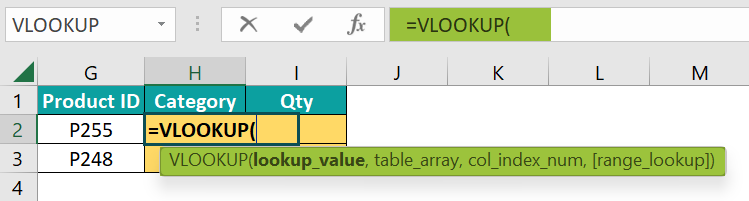2. Choose the lookup value as cell G2 and make the column reference absolute by pressing the F4 key thrice.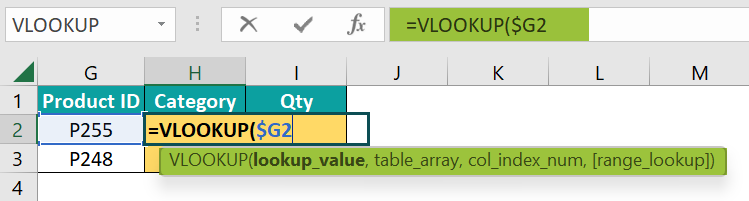3. Choose the table array from cell range A2:E10 and make it as an absolute reference by pressing the F4 key once.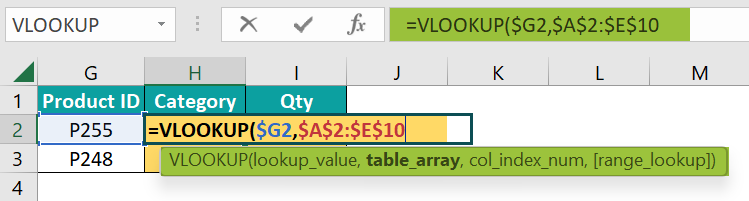Selecting the range and making them an absolute reference looks manual. Hence, to avoid selection manually, we will create a named range for the table area.

4. Exit the VLOOKUP function by pressing the Esc key.

5. Select the table array range A2:H10 and give a name for this range as ‘Data_Table’.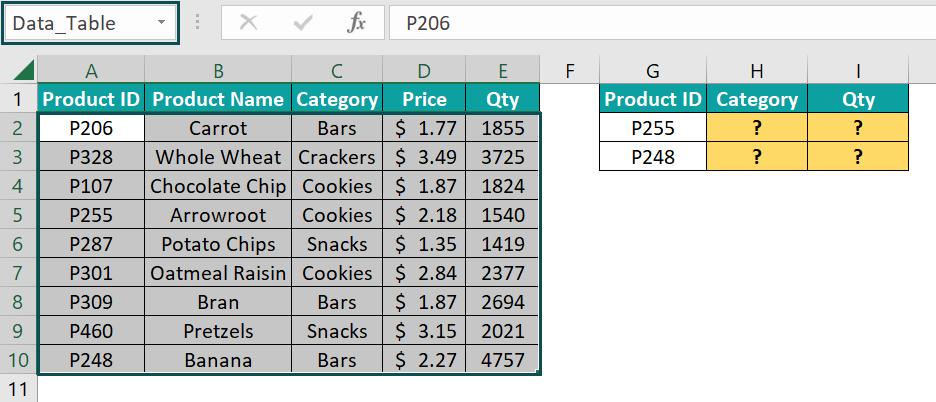Now the range A2:H10 can be referenced by just typing the name “Data_Table”.

6. Again, enter the VLOOKUP function and choose the lookup value as cell G2.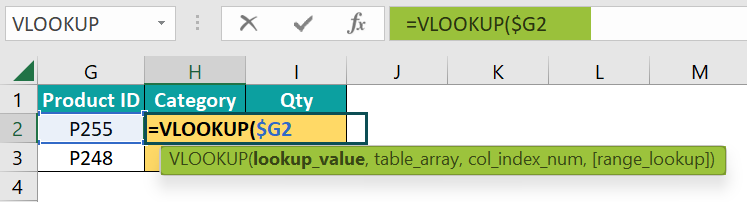7. Now we need to choose the table array, do not manually select the range from A2:E10 rather, just type the name “Data_Table”.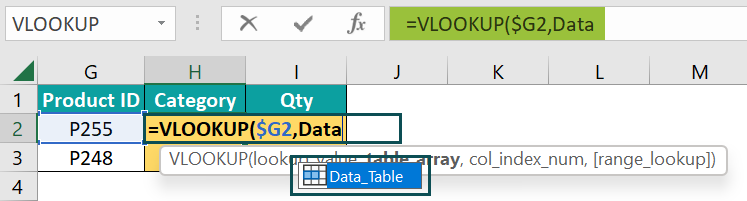The moment we type the name, the list shows the matching results. Choose the “Data_Table” name.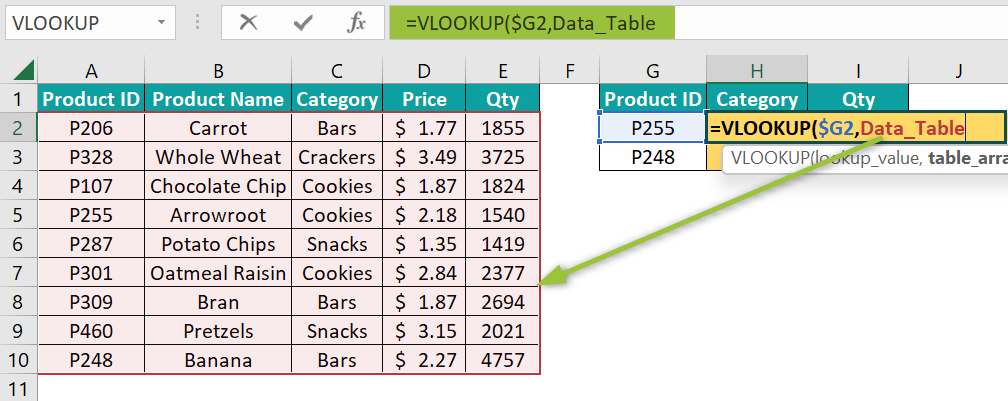As we can see, the moment we choose the named range, it highlights the named area in the same color as the name i.e., Red.

When we use the named range, we need not worry about the absolute reference.

8. Enter the column index number as 3 to get the category name.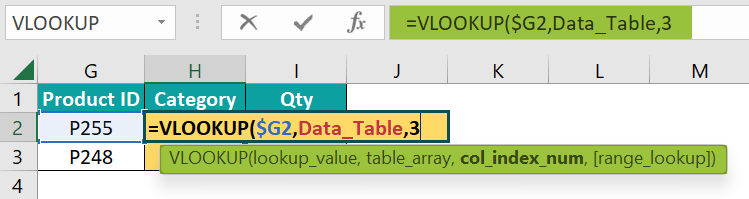9. For the last argument, enter the range lookup as ‘False’ or ‘0’ to get the exact match.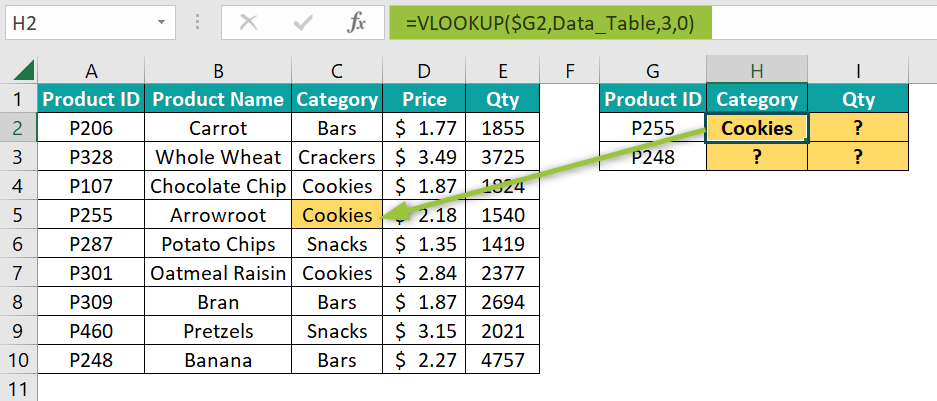10. Copy the formula to the below cell to get the category for the below product ID as well.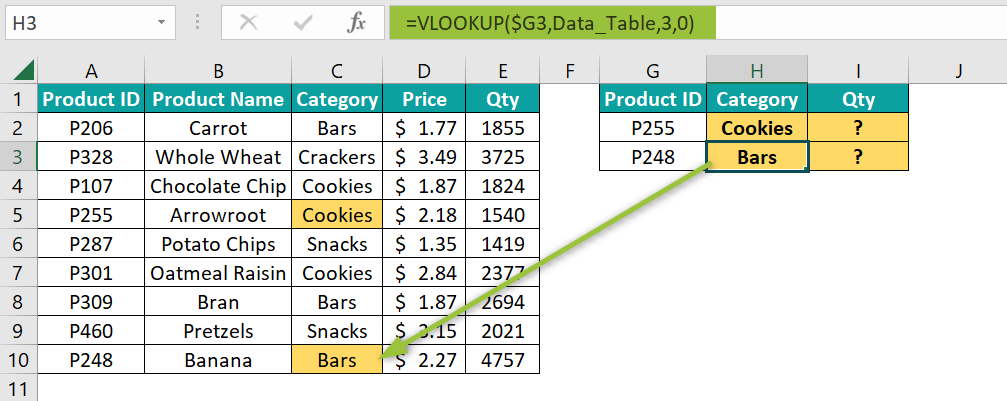11. To get the “Qty” column result, copy the same formula to cell I2 and change the column index number to 5.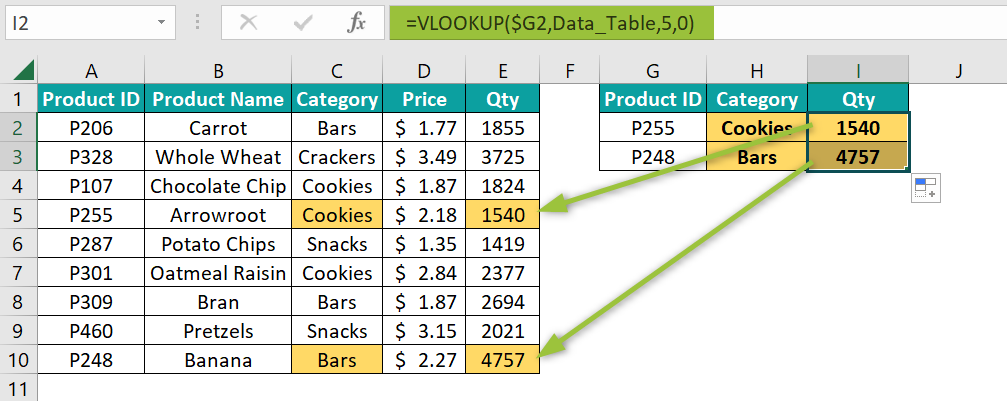By just changing the column index number, we will have the result.

### Examples

#### Example #1 – Create A Named Range To Get The Range Reference From Another Worksheet

Named Ranges will come in handy when we try to retrieve the value from one worksheet to another. Let us look at one such example here.

We have the following data in the worksheet called “Employee_Master”.

Now we have some employee data in another worksheet called “Salary”.

Now we need to fetch salary information for the above 3 employees in the worksheet “Salary” from the “Employee_Master” worksheet.

• Step 1: Enter the VLOOKUP function in cell B2 of the “Salary” worksheet.
• Step 2: Choose the lookup value as cell A2.
• Step 3: Now, we need to choose the table from which we are trying to retrieve the salary information. To choose the table array, we need to switch to the worksheet “Employee_Master” and then choose the range of cells from A2:E10.

However, this switching of worksheets is not a good idea. To work efficiently and super-fast, we need to use named ranges.

• Step 4: Exit the VLOOKUP mode. Choose the range of cells from A2:E10 in the “Employee_Master” worksheet and give a name as “Employee_List”.
• Step 5: Now, in the “Salary” worksheet, enter the VLOOKUP function and give the lookup value as cell A2.
• Step 6: Now, to choose the table array, we need not have to go to the “Employee_Master” worksheet; rather, just type the name that we have given in the previous step.
• Step 7: Enter the column index number as 5.
• Step 8: Enter the range lookup as “False” or 0 to get the exact match.

There we go. We have retrieved the salary information from the worksheet “Employee_Master” without getting the reference from that sheet.

#### Example #2 – Create Dynamic Named Range To Avoid Mistakes

Continuing from Example #1, we will elaborate on the need for dynamic ranges in Excel. For instance, let us add one more employee to the “Employee_Master” worksheet.

We have added a new employee in row number 11.

Let us add the same employee ID in the salary sheet as well to retrieve the salary details.

Using the same formula, we are trying to retrieve the salary information. However, we ended up getting the #N/A error.

The reason for this is the existing named range “Employee_List” referenced to the range of cells from A2:H10 only. Anything added after this range will not be a part of the named range, so the current named range fails to take the new additional data dynamically.

Let us make the existing name range “Employee_List” dynamic by using the following steps.

• Step 2: The Name Manager window opens up. Select the name “Employee_List” and then click on the Edit button.
• Step 3: The Edit Name window opens up. In the Refers to box, enter the following formula.

=OFFSET(Employee_Master!\$A\$2,0,0,COUNTA(Employee_Master!\$A\$2:\$A\$1048576), COUNTA(Employee_Master!\$2:\$2))

• Step 4: Click OK, and the dynamic named range is ready.

Now, we can see the result for the newly added employee ID in the worksheet “Salary”.

Formula Explanation: The formula used to make the named range dynamic is below:

=OFFSET(Employee_Master!\$A\$2,0,0,COUNTA(Employee_Master!\$A\$2:\$A\$1048576), COUNTA(Employee_Master!\$2:\$2))

=OFFSET(Employee_Master!\$A\$2,0,0, = OFFSET Excel function starts from cell A2; it moves down zero rows and moves right zero columns.

COUNTA(Employee_Master!\$A\$2:\$A\$1048576) = COUNTA Excel function will return how many rows are filled up in column A, and the OFFSET function will offset those many rows.

COUNTA(Employee_Master!\$2:\$2) = COUNTA function will return how many columns are filled up in row number 1, and the OFFSET function will offset those many columns.

Like this, we can create a dynamic named range to make life easy and work efficiently with the VLOOKUP function.

### Important Things To Note

• The named Range name should be unique, and there should not be any duplicates.
• The name should not contain any space characters or special characters except underscore (_), and the name should not start with a numerical value.
• The named range scope should be “Workbook” to use the name anywhere within the workbook.
• The VLOOKUP function takes does not require the absolute reference in excel because the named range itself is an absolute reference.
• No need to include the table header while naming the range.

1. How to VLOOKUP Names in Excel?

Whenever we apply the VLOOKUP function in Excel, we need to reference the table array, and this table array is often selected manually.

For instance, look at the following data in Excel.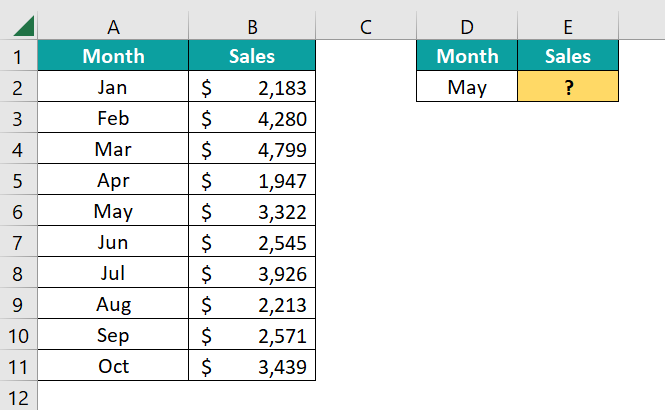We have monthly sales from A2:B11, and in cell E2 we are trying to fetch the value for the month “May”.

When we give a table array, we need to choose the cells from A2:B11. Instead, we can give a name.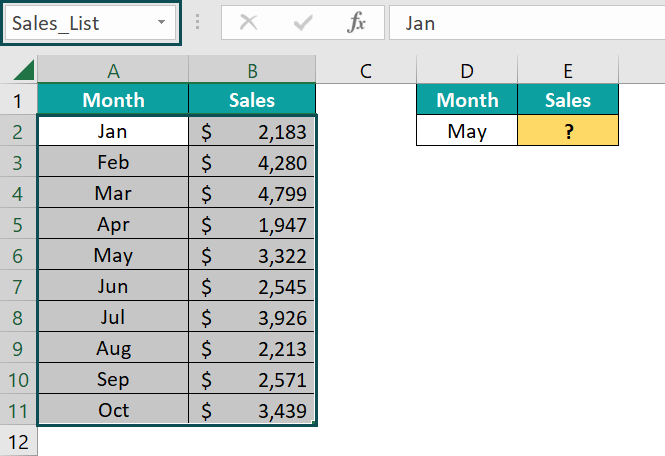By selecting the range of cells A2:B11, we have given a name as ‘Sales_List’ and this name can be used to reference range A2:B11.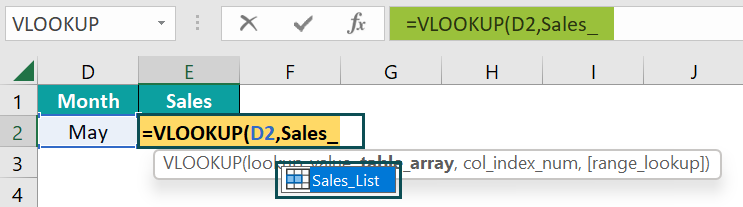As we can see, instead of giving the cell range A2:B11, we have given the name range as “Sales_List”.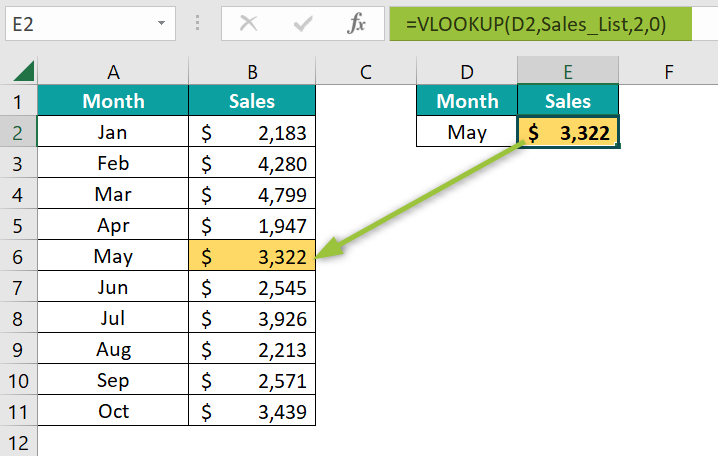2. Why are VLOOKUP Names Not Working?

The first thing is data should be vertically arranged, and result columns should be to the right of the lookup value column.

The second thing is the named range should be present in the worksheet.

If any of these two is missing, then our names will not work with the VLOOKUP function.

3. What are the 4 arguments you give in VLOOKUP names?

The 4 arguments of the VLOOKUP function are: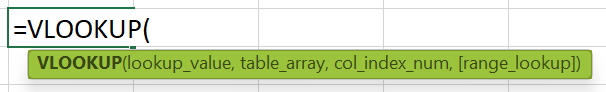• Lookup Value
• Table Array
• Column Index Number
• Range Lookup